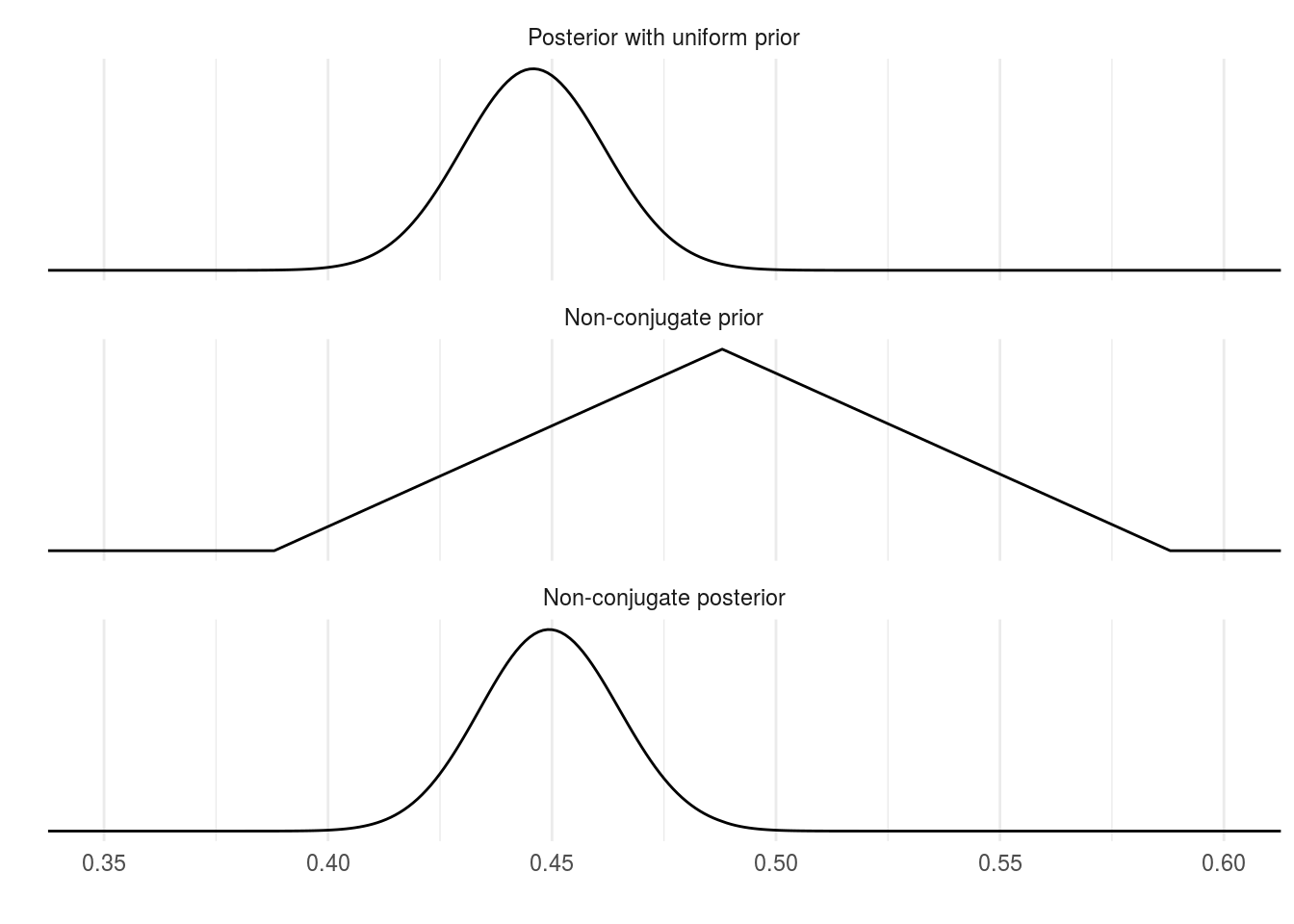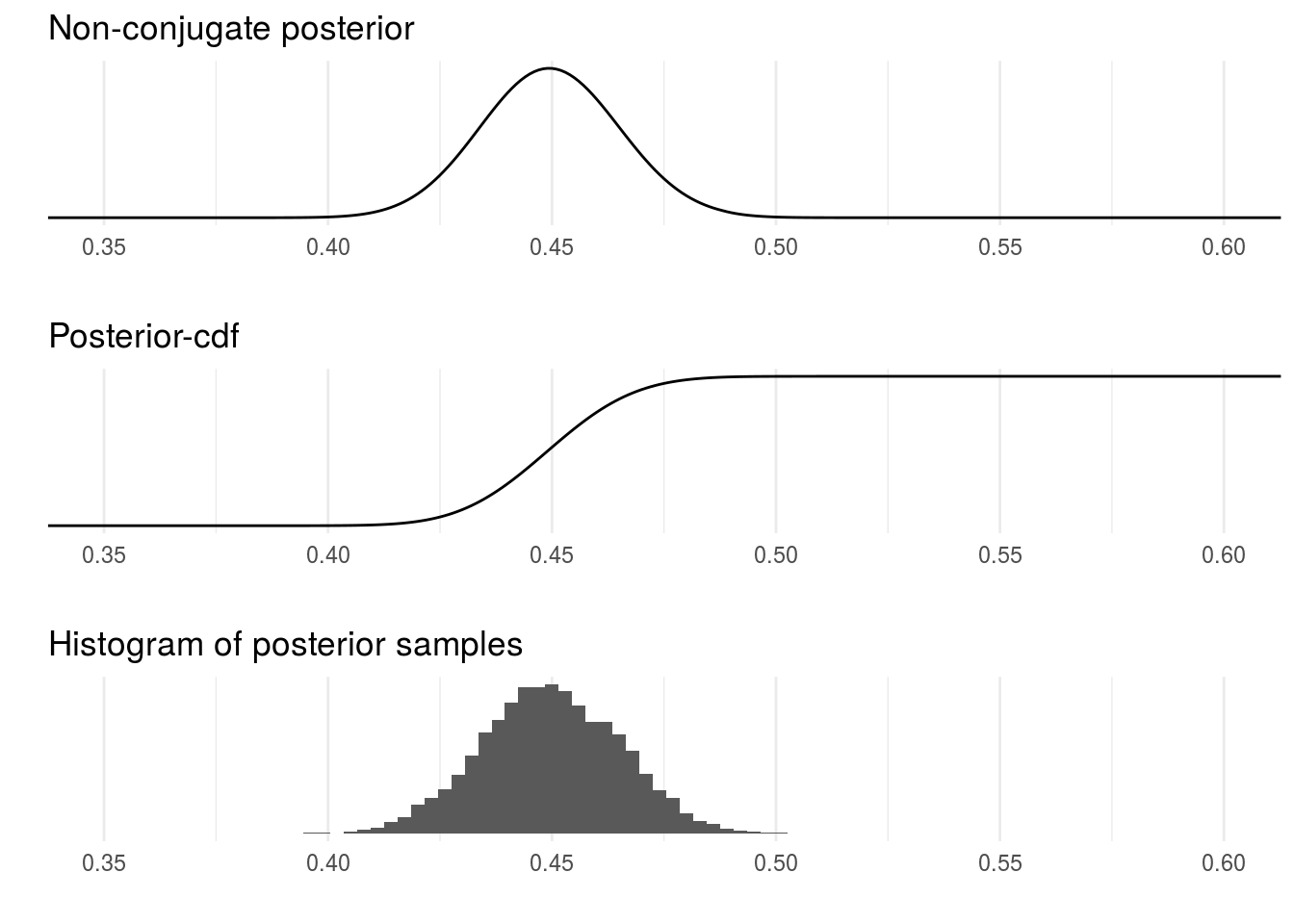## Probability of a girl birth given placenta previa (BDA3 p. 37).

Calculate the posterior distribution on a discrete grid of points by multiplying the likelihood and a non-conjugate prior at each point, and normalizing over the points. Simulate samples from the resulting non-standard posterior distribution using inverse cdf using the discrete grid.

ggplot2 and gridExtra are used for plotting, tidyr for manipulating data frames

library(ggplot2)
theme_set(theme_minimal())
library(gridExtra)
library(tidyr)

### Evaluating posterior with non-conjugate prior in grid

Posterior with observations (437,543) and uniform prior (Beta(1,1))

a <- 437
b <- 543

Evaluate densities at evenly spaced points between 0.1 and 1

df1 <- data.frame(theta = seq(0.1, 1, 0.001))
df1$con <- dbeta(df1$theta, a, b)

Compute the density of non-conjugate prior in discrete points, i.e. in a grid this non-conjugate prior is the same as in figure 2.4 in the book

pp <- rep(1, nrow(df1))
pi <- sapply(c(0.388, 0.488, 0.588), function(pi) which(df1$theta == pi)) pm <- 11 pp[pi:pi] <- seq(1, pm, length.out = length(pi:pi)) pp[pi:pi] <- seq(1, pm, length.out = length(pi:pi)) normalize the prior df1$nc_p <- pp / sum(pp)

compute the un-normalized non-conjugate posterior in a grid

po <- dbeta(df1$theta, a, b) * pp normalize the posterior df1$nc_po <- po / sum(po)

Plot posterior with uniform prior, non-conjugate prior and the corresponding non-conjugate posterior

# gather the data frame into key-value pairs
# and change variable names for plotting
df2 <- gather(df1, grp, p, -theta, factor_key = T) #%>%
levels(df2$grp) <- c('Posterior with uniform prior', 'Non-conjugate prior', 'Non-conjugate posterior') ggplot(data = df2) + geom_line(aes(theta, p)) + facet_wrap(~grp, ncol = 1, scales = 'free_y') + coord_cartesian(xlim = c(0.35,0.6)) + scale_y_continuous(breaks=NULL) + labs(x = '', y = '')### Inverse cdf sampling compute the cumulative density in a grid df1$cs_po <- cumsum(df1$nc_po) Sample from uniform distribution U(0,1) # set.seed(seed) is used to set seed for the randon number generator set.seed(2601) # runif(k) returns k uniform random numbers from interval [0,1] r <- runif(10000) Inverse-cdf sampling # function to find the value smallest value theta at which the cumulative # sum of the posterior densities is greater than r. invcdf <- function(r, df) df$theta[sum(df\$cs_po < r) + 1]
# sapply function for each sample r. The returned values s are now
# random draws from the distribution.
s <- sapply(r, invcdf, df1)

Create three plots: p1 is the posterior, p2 is the cdf of the posterior and p3 is the histogram of posterior samples (drawn using inv-cdf)

p1 <- ggplot(data = df1) +
geom_line(aes(theta, nc_po)) +
coord_cartesian(xlim = c(0.35, 0.6)) +
labs(title = 'Non-conjugate posterior', x = '', y = '') +
scale_y_continuous(breaks = NULL)
p2 <- ggplot(data = df1) +
geom_line(aes(theta, cs_po)) +
coord_cartesian(xlim = c(0.35, 0.6)) +
labs(title = 'Posterior-cdf', x = '', y = '') +
scale_y_continuous(breaks = NULL)
p3 <- ggplot() +
geom_histogram(aes(s), binwidth = 0.003) +
coord_cartesian(xlim = c(0.35, 0.6)) +
labs(title = 'Histogram of posterior samples', x = '', y = '') +
scale_y_continuous(breaks = NULL)
# combine the plots
grid.arrange(p1, p2, p3)